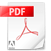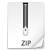# mKR: A Code for Convex Quadratic Programs with Box-Constraints

We suggest a primal-dual active set method for the convex quadratic optimization problem

min J(x),
subject to a ≤ x ≤ b,
where J(x) = x' Qx + q x,

Q is a positive-definite n×n matrix, and b, q ∈ R^n . This problem is well understood from a theoretical point of view with global optimality characterized by the Karush-Kuhn-Tucker conditions. The problem appears as a basic building block in many applications, for instance in the context of sequential quadratic optimization methods to solve more general nonlinear problems. It also appears in problems from mathematical physics.

Our method (for more details see our preprint) extends the infeasible active set approach of Kunisch and Rendl [K. Kunisch and F. Rendl. An infeasible active set method for convex problems with simple bounds. SIAM Journal on Optimization, 14(1):35-52, 2003.] Based on a guess on the active set, a primal-dual pair (x,α) is computed that satisfies stationarity and the complementary condition. If x is not feasible, the variables connected to the infeasibilities are added to the active set and a new primal-dual pair (x,α) is computed. This process is iterated until a primal feasible solution is generated. Then a new active set is determined based on the feasibility information of the dual variable α. Strict convexity of the quadratic problem is sufficient for the algorithm to stop after a finite number of steps with an optimal solution. Computational experience indicates that this approach also performs well in practice.

The corresponding paper "A Feasible Active Set Method for Strictly Convex Quadratic Problems with Simple Bounds" appeared in SIAM Journal of Optimimization 25-3 (2015), pp. 1660-1685. Here you can download a preprint of this paper:Preprint_mKR
cqp_with_box_oo.pdf

In the qp_code_mkr.zip-file below you find the Kunish-Rendl-Algorithm and the modified Kunish-Rendl-Algorithm for both one-sided bounds and box-constraints. Additionally we provide files reproducing the experiments depicted in the mkr.pdf-preprint above. The calls for reproducing the experiments are denoted as the corresponding tables in the preprint:

Table_6_1

Table_6_2

Tables_6_3_and_6_4

Tables_6_5_and_6_6

Table_6_7

Table_6_8

Table_6_9

Table_6_10qp_code_mkr_preprint.zip
Compressed Archive in ZIP Format 36.9 KB

Note: To call "Table_6_2.m" and "Table_6_9.m" you have to possess CPLEX and connect your matlab and CPLEX codes. For more details see here.